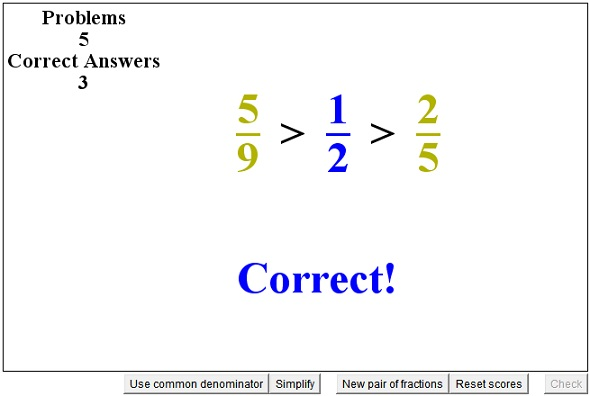# Fraction Comparison Sped up

There are ways and ways to compare fractions. As with operations on integers, there is a multitude of shortcuts for fraction comparison.

(I shall always assume that both the numerators and denominators of the fractions below are positive integers.)

The standard way to compare two fractions a/c and b/d is by cross-multiplication:

a/c > b/d if and only if ad > bc.

However, quite often computing the products is cumbersome and is actually unnecessary. In many circumstances the result can be obtained at a glance.

1. Assume a > b. Then

a/c > b/c

This is because one of possible readings of fraction a/b is "a divided by b" or "a b-ths" so that the greater is a the more "b-ths" are signified by a/b. In particular,

(a + 1)/c > a/c and a/c > (a - 1)/c.

For example, 4/5 > 3/5 and 7/12 > 5/12.

2. Assume c < d, then

a/c > a/d.

In particular,

a/c > a/(c + 1) and a/(c - 1) > a/c.

This is because the greater is c, the smaller is its reciprocal 1/c.

For example, 5/4 < 5/6 simply because 4 > 6.

3. In the last example, 5/4 is an improper fractions, meaning that its numerator is greater than the denominators which, in turn, implies that the fraction exceeds 1. On the other hand, 5/6 is less than 1: 5/4 > 1 > 5/6. By transitivity of the symbol of inequality, 5/4 > 5/6.

The trick here is to find a fraction - the simpler the better - that is obviously between the two given fractions. Say, you have to compare 7/12 and 4/9. 7/12 > 6/12 = 1/2, while 4/9 = 8/18 < 9/18 = 1/2. We see that 7/12 > 1/2 > 4/9 and, therefore, 7/12 > 4/9.

4. For proper fractions a/c, i.e., for a/c < 1,

a/c < (a + 1)/(c + 1) and (a - 1)/(c - 1) < a/c.

The opposite is true for the improper fractions: if a/c > 1 then

a/c > (a + 1)/(c + 1) and (a - 1)/(c - 1) > a/c.

For example, 7/6 > 8/7 > 9/8 > 10/9, etc., because, as we progress to the right, we add smaller and smaller fraction to 1.

5. The mediant fraction (a + b)/(c + d) is always between the given two, a/c and b/d. Sometimes, it could be more convenient to compare each of the given fractions to their mediant than to compare them between themselves.

A very simple example supports rule #3. For example, 5/4 > 4/5 because 5/4 > (5 + 4)/(4 + 5) > 4/5.

Sometimes, a combination of rules works best. For example, compare 8/7 and 9/5: 8/7 < 9/7 < 9/5 so that 8/7 < 9/5 without much ado.

The applet below furnishes a tool to practice fraction comparison. It displays three fractions of which the middle one is modifiable. You can change the numerator and the denominator independently by clicking a little off the vertical central line. When satisfied, click on the "Check" button at the lower right corner of the applet.

### If you are reading this, your browser is not set to run Java applets. Try IE11 or Safari and declare the site https:///www.cut-the-knot.org as trusted in the Java setup.What if applet does not run?### Fractions